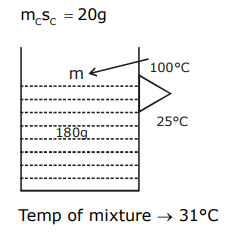# A calorimeter of water equivalent 20 g contains 180 g of water at 25°C.

A calorimeter of water equivalent 20 g contains 180 g of water at 25°C. ‘m’ grams of steam at 100°C is mixed in it till the temperature of the mixture is 31°C. The value of ‘m’ is close to (Latent heat of water = 540 cal g–1, specific heat of water = 1 cal g–1 °C–1)

1) 2

2) 2.6

3) 4

4) 3.2Heat lost by steam = heat gained by water

180×1×(31-25)+20×(31-25)= m×540+m×1×(100-31)

180×6+20×6 = 540m + 100 m – 31m

1080+120 = 640 m – 31m

1200 = 609m

m = 1200/609

= 1.97

m = 2(1)(1)